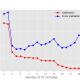###### Principal Component Regression in Python
05/12/2018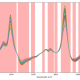###### A variable selection method for PLS in Python
07/04/2018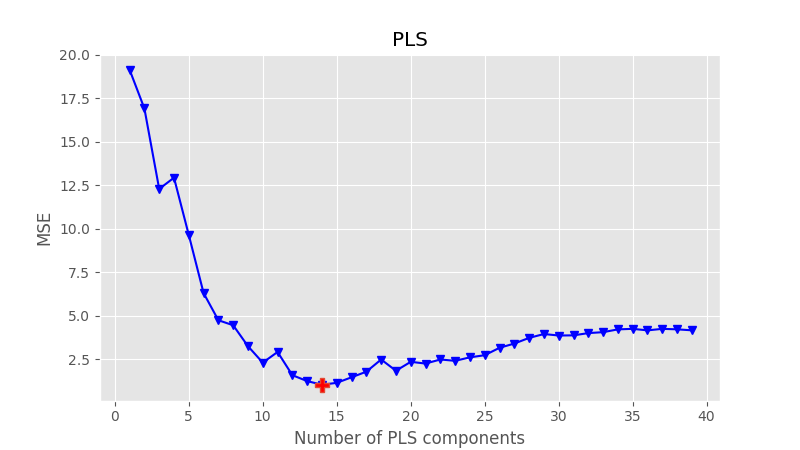Hi everyone, and thanks for stopping by. Today we are going to present a worked example of Partial Least Squares Regression in Python on real world NIR data.

PLS, acronym of Partial Least Squares, is a widespread regression technique used to analyse near-infrared spectroscopy data. If you know a bit about NIR spectroscopy, you sure know very well that NIR is a secondary method and NIR data needs to be calibrated against primary reference data of the parameter one seeks to measure. This calibration must be done the first time only. Once the calibration is done, and is robust, one can go ahead and use NIR data to predict values of the parameter of interest.

In previous posts we discussed many times qualitative analysis of NIR data by Principal Component Analysis (PCA), and how one can make a step further and build a regression model using Principal Component Regression (PCR).

PCR is quite simply a regression model built using a number of principal components derived using PCA. In our last post on PCR, we discussed how PCR is nice and simple, but limited by the fact that it does not take into account anything other than the regression data. That is, our primary reference data are not considered when building a PCR model. That is obviously not optimal, and PLS is a way to fix that.

In this post I am going to show you how to build a simple regression model using PLS in Python. This is the overview what we are going to do.

1. Mathematical introduction on the difference between PCR and PLS regression (for the bravest)
2. Present the basic code for PLS
3. Discuss the data we want to analyse and the pre-processing required. Spoiler: we’ll use NIR spectra of rice with each spectrum having an associated value of amylose content, that is the quantity we want to calibrate for.
4. Since the data is divided into a calibration and a validation set, we will build our model using the calibration data only, and then test its performance on the validation set.

# Difference between PCR and PLS regression

Before working on some code, let’s very briefly discuss the mathematical difference between PCR and PLS. I decided to include this description because it may be of interest for some of our readers, however this is not required to understand the code. Feel free to skip this section altogether if you’re not feeling like dealing with math right now. I won’t hold it against you.

Still here? OK here we go.

Both PLS and PCR perform multiple linear regression, that is they build a linear model, $$Y=XB+E$$. Using a common language in statistics, $$X$$ is the predictor and $$Y$$ is the response. In NIR analysis, $$X$$ is the set of spectra, $$Y$$ is the quantity – or quantities- we want to calibrate for (in our case the amylose values). Finally $$E$$ is an error.

As we discussed in the PCR post, the matrix $$X$$ contains highly correlated data and this correlation (unrelated to amylose) may obscure the variations we want to measure, that is the variations of the amylose content. Both PCR and PLS will get rid of the correlation.

In PCR (if you’re tuning in now, that is Principal Component Regression) the set of measurements $$X$$ is transformed into an equivalent set $$X’=XW$$ by a linear transformation $$W$$, such that all the new ‘spectra’ (which are the principal components) are linearly independent. In statistics $$X’$$ is called the factor scores. The linear transformation in PCR is such that it minimises the covariance between the different rows of $$X’$$. That means this process only uses the spectral data, not the response values.

This is the key difference with between PCR and PLS regression. PLS is based on finding a similar linear transformation, but accomplishes the same task by maximising the covariance between $$Y$$ and $$X’$$. In other words, PLS takes into account both spectra and response values and in doing so will improve on some of the limitations on PCR. For these reasons PLS is one of the staples of modern chemometrics.

I’m not sure if that makes any sense to you, but that was my best shot at explaining the difference without writing down too many equations.

# PLS Python code

OK, here’s the basic code to run PLS, based on Python 3.5.2.

As you can see, sklearn has already got a PLS package, so we go ahead and use it without reinventing the wheel. So, first we define the number of components we want to keep in our PLS regression. I decided to keep 5 components in this case, but later will use that as a free parameter to be optimised. Once the PLS object is defined, we run the regression on the calibration data X_calib (the predictor) and Y_calib (the known response). The third step is to use the model we just built to predict the response of the validation data X_valid. The important bit is that the validation data has not been used to build the model, so the validation test acts as a genuine check of the robustness of our calibration.

To check how good our calibration is, we measure the usual metrics (see PCR post for more details on it). We’ll evaluate these metric by comparing the result of the prediction, Y_pred with the known responses Y_valid. To optimise the parameters of our PLS regression (for instance pre-processing steps and number of components) we’ll just track those metrics, most typically the MSE.

One more thing. In the actual code the various X_calib, Y_calib, etc. are numpy array read from a spreadsheet. For that you’ll probably need to import numpy (of course), pandas and a bunch of other libraries which we will see below.

This is the basic block of PLS regression in Python. You can take this snippet and use it in your code, provided that you have defined the arrays in the right way. Now it’s time for us to take a look at the data import and pre-processing.

# NIR data import and pre-processing

Here’s the complete list of imports

Next let’s import the data, which is kept into an Excel spreadsheet. The data is composed of 516 spectra of rice. Each spectrum has a corresponding amylose value (the response) associated with it. Of these spectra, the first 422 are the calibration values, and the rest is the validation set. Finally, each spectrum is taken over 100 wavelength points, from 850 nm to 1048 nm.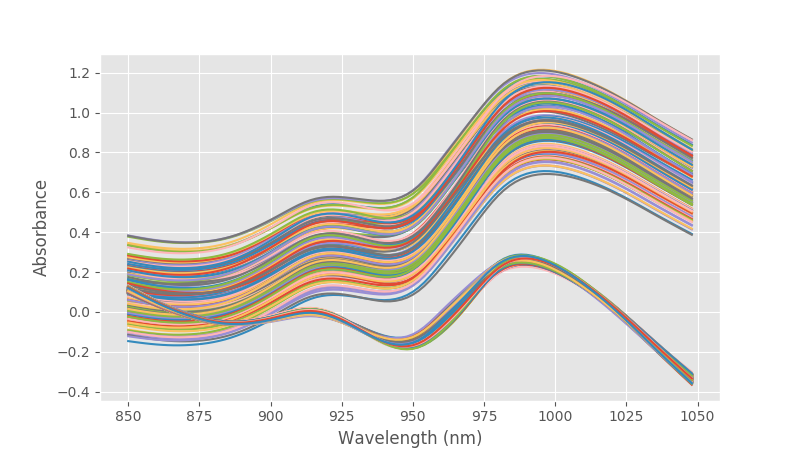Whoa, something’s unusual with these data. In this case an unavoidable instrumental difference is present between two sets of data. The data were acquired in different moments from different varieties of rice, and in one case there was some instrumental systematic error. This errors shows up as baseline error plus a dispersion of the data. Data can be easily sortedby PCA (we shall see how to do that in the future) and corrected with multiplicative scatter correction (also for the future), however a simple yet effective way to get rid of baseline and linear variations is to perform second derivative on the data.  Let’s do that and check the result.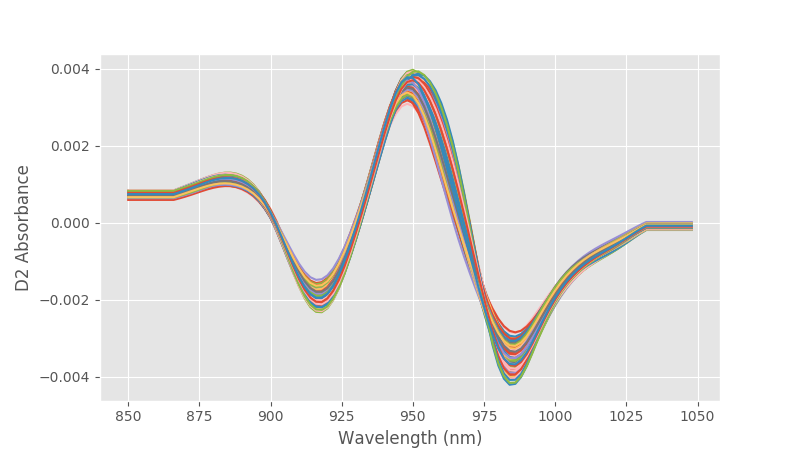The offset is gone and the data look more bunched together. For this example that will do.

Now it’s time to get to the optimisation of the PLS regression. As anticipated above, we want to run a PLS regression with a variable number of components and test its performance on the validation set. In practice we want to find the number of components that minimises the MSE. Let’s write a function for it

This functions first runs a loop over the number of PLS components (up to 40) and calculate the MSE of prediction.  Second it finds the number of components that minimises the MSE ans uses that value to run PLS again. The second time a whole bunch of metrics is calculated and printed. To run the function we simply type:

The first plot that’ll come up is the MSE as a function of the number of components. The suggested number of components data point is highlighted and printed on screen.The second plot is the actual regression figure, including the metrics for the prediction.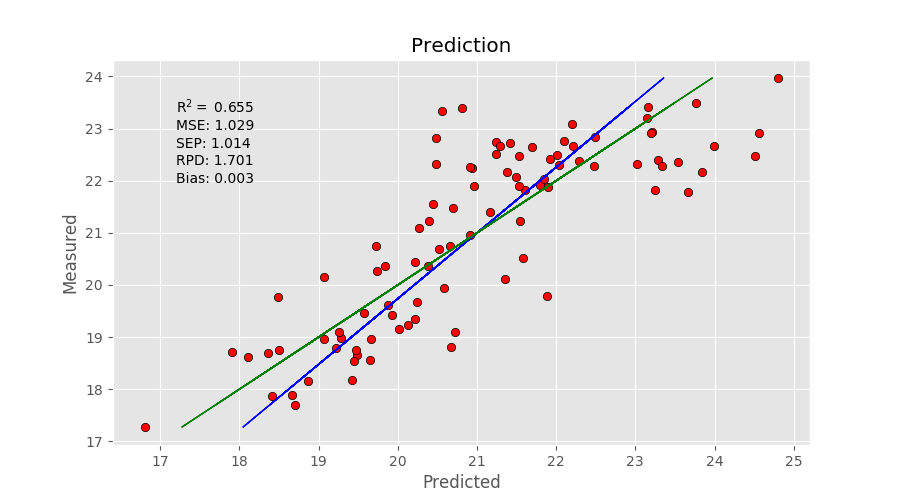At the same time, the following information is going to be printed on screen.
 Suggested number of components: 14

R2: 0.655
MSE: 1.029
SEP: 1.014
RPD: 1.701
Bias: 0.003

Well, we reached the end of this introductory post on PLS regression using Python. I hope you enjoyed reading it and I’ll see you next time. Thanks again for reading!##### Daniel Pelliccia
Physicist and an entrepreneur. Founder and Managing Director at Instruments & Data Tools, specialising in optical design and analytical instrumentation. Founder at Rubens Technologies, the intelligence system for the fresh fruit export industry.